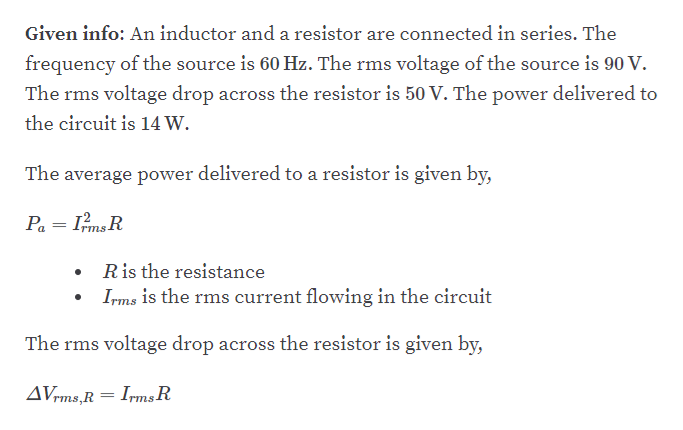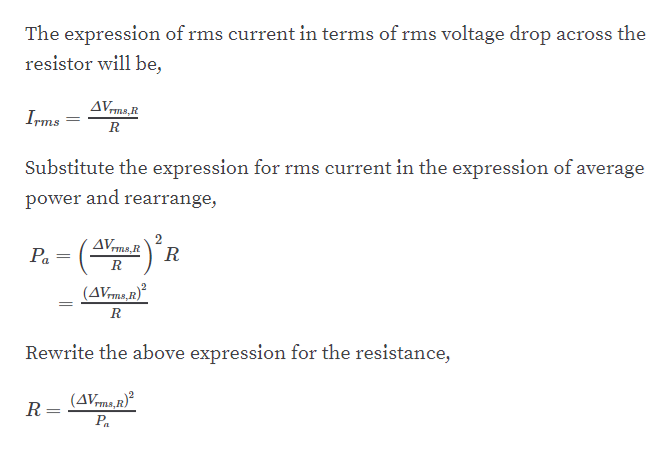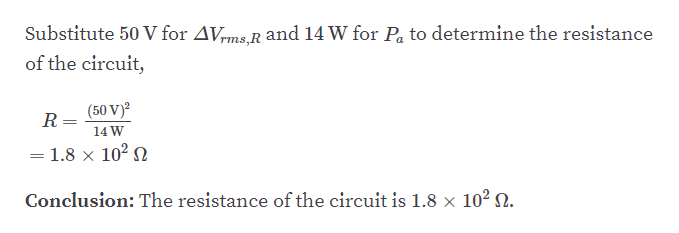# An inductor and a resistor are connected in series. Whenconnected to a 60. - Hz, 90. - V (rms) source, the voltage dropacross the resistor is found to be 50. V (rms) and the powerdelivered to the circuit is 14 W. Find (a) the value of theresistance and (b) the value of the inductance.

Question
2 views

An inductor and a resistor are connected in series. When
connected to a 60. - Hz, 90. - V (rms) source, the voltage drop
across the resistor is found to be 50. V (rms) and the power
delivered to the circuit is 14 W. Find (a) the value of the
resistance and (b) the value of the inductance.

check_circle

Step 1

part A :help_outlineImage TranscriptioncloseGiven info: An inductor and a resistor are connected in series. The frequency of the source is 60 Hz. The rms voltage of the source is 90 V. The rms voltage drop across the resistor is 50 V. The power delivered to the circuit is 14W. The average power delivered to a resistor is given by, Pa = ImsR Ris the resistance Irms is the rms current flowing in the circuit The rms voltage drop across the resistor is given by, AVrms,R = IrmsR fullscreen
Step 2help_outlineImage TranscriptioncloseThe expression of rms current in terms of rms voltage drop across the resistor will be, AVms,R TRS Irms Substitute the expression for rms current in the expression of average power and rearrange, AVms,R Pa = ( R (AVrms,R)² %3D Rewrite the above expression for the resistance, (AVms,r) R= Pa fullscreen
Step 3help_outlineImage TranscriptioncloseSubstitute 50 V for AVms R and 14 W for Pa to determine the resistance of the circuit, (50 V)? R = 14 W = 1.8 × 10² N Conclusion: The resistance of the circuit is 1.8 × 10² N. fullscreen

### Want to see the full answer?

See Solution

#### Want to see this answer and more?

Solutions are written by subject experts who are available 24/7. Questions are typically answered within 1 hour.*

See Solution
*Response times may vary by subject and question.
Tagged in

### Other